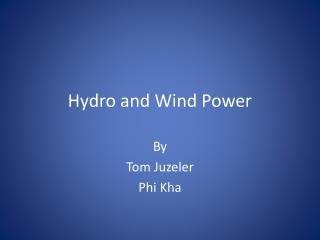Download PresentationHydro and Wind Power

# Hydro and Wind Power

Download Presentation## Hydro and Wind Power

- - - - - - - - - - - - - - - - - - - - - - - - - - - E N D - - - - - - - - - - - - - - - - - - - - - - - - - - -
##### Presentation Transcript

1. Hydro and Wind Power By Tom Juzeler Phi Kha

2. HYDRO POWER • Hydro Power is basically the power derived from the movement of water. • Hydroelectricity comes from the damming of the rivers as they flow to the ocean and then releasing the water to turn turbines connected to electric generators.

3. Advantages of Hydropower • No polluting emission, • No waste heat rejected as thermal pollution, • Hydroelectric facilities have a lifespan that lasts for decades, • Small maintenance requirements, • Other uses of dam: flood control, irrigation, supply source of drinking water, and recreational opportunities.

4. Disadvantages of Hydropower • Loss of free flowing streams, • Loss of land flooded by reservoirs, • Disturbance to aquatic life (salmon), • Silt buildup, • Potential consequences of dam failure.

5. WIND POWER • Wind power is the kinetic energy from the wind that can be converted into a useful type of energy.

6. Relationship of power and velocity • Under average conditions • P/m2 = 6.1 x 10-4 v3 • Where, P is power of wind in kilowatt, P/m2 is wind power per square meter of cross section • V is wind velocity in meter per second • This is the total power in wind (total kinetic energy), which cannot all be extracted by any practical device.

7. Problems with Wind Power • Costs • Variability of wind speeds • Energy storage • Loud noise of wind turbines • Not a pretty sight.

8. Linfield’s Wind Turbine Proposal • How many 2.5MW GE wind turbines are needed to power Linfield College? • All campus without suburbs, energy needs = 8,751,375 KWh/year = 8.75 MWh/year • Average wind speed for McMinnville = 8 m/s (taken from figure 5.7 with explanation to follow) • Convert the average wind speed of the area into average capacity of a GE wind turbine (using GE graph) = 1500 KWh = 1.5 MWh

9. GE turbine power capacity at certain wind speeds At an average wind speed of 8 m/s in the area, the average power the turbine can produce is 1500 KW

10. The average output of a GE turbine for a year in the McMinnville area: • 1500 KWh x 24 hours x 365 days = 13,140,000 KWh per year. • We need 8,751,375 KWh to power the college for one year. 13,140,000KWh is produced by one wind turbine. Therefore, we only need one wind turbine to supply the college in any given year.

11. Assumptions • Wind turbine is 100% efficient. • Constant wind of 8 m/s year round. • The energy consumption for the college is the same every year as it was in 2007-2008.

12. How to find the average wind speed • There are two ways to figure out the wind speed at 50 meter altitude for McMinnville. • From http://www.nrel.gov/gis/wind.html, there are interactive wind resource maps and a classification of wind speed for the US. We can approximate the data to get the wind speed for McMinnville area. • Alternatively, we can derive the same information from the wind density shown in figure 5.7, as displayed on the next slide.

13. Find the wind speed from wind density • According to figure 5.7 (pg. 139), the average wind density for the McMinnville area is 300 W/m2 = 0.3 kW/m2 • Find the corresponding wind speed by using P/m2 = 6.1 x 10-4 v3 • 0.3 kW/m2 = 6.1 x 10-4 v3, so v3 = 0.3/(6.1 x 10-4) = 491.803 (m/s)3 • V ≈ 8m/s by taking cube root of v3

14. References • GE 2.5 MW wind turbine configuration http://www.gepower.com/prod_serv/products/wind_turbines/en/2xmw/index.htm • Figure 5.7, textbook Energy and Environment • Fiscal year 2007-2008 Linfield Electrical Consumption Comparison. • http://www.nrel.gov/gis/wind.html